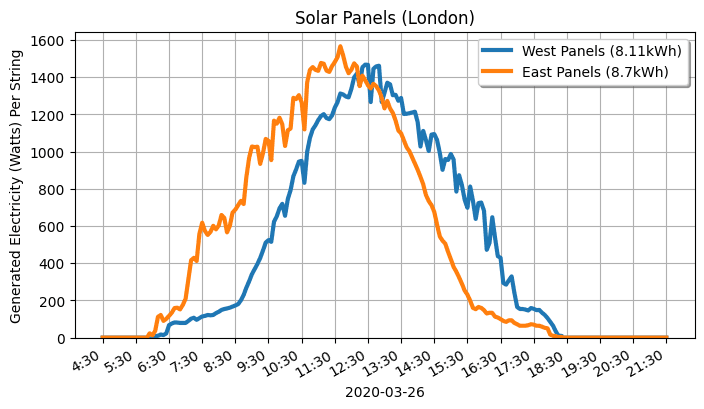# Relaunching @edent_solar. Part 4 - Dual String MPPT APIs

I'm hooking my solar panels up to the Internet!

My solar panels have an API! It tells me the total amount of power they've generated each day. But there's a small problem... I have panels on the East and West sides of my roof. My solar inverter has two MPPT "String" inputs. That is, East and West supply power separately. Luckily, there's an API for that!

Thanks to "Grannos" on the PVOutput forum for their excellent open source code which helped get me started.

## API Call

This is slightly hidden in the official API documentation. You have to query the historic data. If you query today's date, you can get sort-of real time data.

This is the call - replace the IP address with your own:

``````http://192.168.0.123/solar_api/v1/GetArchiveData.cgi?
Scope=System&
StartDate=2020-03-25&
EndDate=2020-03-25&
Channel=Voltage_DC_String_1&
Channel=Current_DC_String_1&
Channel=Voltage_DC_String_2&
Channel=Current_DC_String_2
``````

## Data Structure

This is a truncated view of the data:

``````{
"Body": {
"Data": {
"inverter/1": {
"Data": {
"Current_DC_String_1": {
"Unit": "A",
"Values": {
...
"57000": 3.3999999999999999,
"57300": 2.8300000000000001,
"57600": 2.6400000000000001,
...
},
},
"Current_DC_String_2": {
"Unit": "A",
"Values": {
...
"57000": 0.42999999999999999,
"57300": 0.41999999999999998,
"57600": 0.41000000000000003,
...
},
},
"Voltage_DC_String_1": {
"Unit": "V",
"Values": {
...
"57000": 215.5,
"57300": 233.40000000000001,
"57600": 228.90000000000001,
...
},
},
"Voltage_DC_String_2": {
"Unit": "V",
"Values": {
...
"57000": 271.10000000000002,
"57300": 264.19999999999999,
"57600": 260.5,
...
},
}
},
``````

The timestamps are seconds-since-midnight. They're sampled every 5 minutes (300 seconds) - so `57000` is `15:50`.

## I've got the power!

To get the power, multiply the Amps by the Voltage. That gets you the Watts. SCIENCE!

I'm sure there is a better way to do this, and I'd be grateful for any pointers.

### Get today's data from the inverter

``````today = now.strftime("%Y-%m-%d")

# Get today's data from the inverter
API_url = "http://" + fronius_IP_address + "/solar_api/v1/GetArchiveData.cgi?Scope=System&StartDate=" + today + "&EndDate=" + today + "&Channel=Voltage_DC_String_1&Channel=Current_DC_String_1&Channel=Voltage_DC_String_2&Channel=Current_DC_String_2"

response = requests.get(url=API_url)
``````

### Add it into a structured and ordered format

``````string1_current = data["Body"]["Data"]["inverter/1"]["Data"]["Current_DC_String_1"]["Values"]
#  Keys to ints, Values to floats
string1_current = {int(k):float(v) for k,v in string1_current.items()}
string1_current = sorted(string1_current.items())

string2_current = data["Body"]["Data"]["inverter/1"]["Data"]["Current_DC_String_2"]["Values"]
#  Keys to ints, Values to floats
string2_current = {int(k):float(v) for k,v in string2_current.items()}
string2_current = sorted(string2_current.items())

string1_voltage = data["Body"]["Data"]["inverter/1"]["Data"]["Voltage_DC_String_1"]["Values"]
#  Keys to ints, Values to floats
string1_voltage = {int(k):float(v) for k,v in string1_voltage.items()}
string1_voltage = sorted(string1_voltage.items())

string2_voltage = data["Body"]["Data"]["inverter/1"]["Data"]["Voltage_DC_String_2"]["Values"]
#  Keys to ints, Values to floats
string2_voltage = {int(k):float(v) for k,v in string2_voltage.items()}
string2_voltage = sorted(string2_voltage.items())
``````

### Calculating the power

``````timestamp_list = []
string1_watts  = []
string2_watts  = []

for current, voltage in zip(string1_current, string1_voltage):
timestamp_list.append(str(datetime.timedelta(seconds=current))[:-3]) #   Remove the seconds
string1_watts.append(int(current * voltage))

for current, voltage in zip(string2_current, string2_voltage):
string2_watts.append(int(current * voltage))

#  Remove the first 4:30 hours (54 * 5 minutes)
timestamp_list = timestamp_list[54:]
string1_watts  = string1_watts[54:]
string2_watts  = string2_watts[54:]

#  Total Power Generation
#  Bit sketchy. Only samples ever 5 minutes.
string1_kWh = str(round(sum(string1_watts) * 5 / 60 / 1000, 2))
string2_kWh = str(round(sum(string2_watts) * 5 / 60 / 1000, 2))
``````

### Write the CSV

This is my least favourite part of the code. It zips together the timestamps and the wattage from the first String. Then zips it with the 2nd String.

``````with open(csv_file, 'w', newline='') as csvfile:
writer = csv.writer(csvfile)
for a, b in zip(zip(timestamp_list,string1_watts),string2_watts):
t  = str(a)
w1 = str(a)
w2 = str(b)
writer.writerow([t,w1,w2])
``````

## Source Code

All available on my GitHub

## Output